Fluids Index

Dimensional Analysis

Introduction..... Symbols...... Types of Similarity...... Simple example...... Buckinghams theorem......

Introduction

Many physical relationships in engineering and especially in fluid mechanics are, by nature, extremely complex.   Often a phenomenon is too complicated to, theoretically, derive a formula describing it e.g the forces experienced when an object moves through a fluid.   Dimensional analysis is then used to identify variables which can be combined in groups which are definitely related.  Experiments can then be completed to formulate this relationship and allow determination of the actual performance characteristics of real world systems..

This method derives from the principle that each term in an equation depicting a physical relationship must have the same dimension.   Non-dimensional quantities expressing the relationship among the variables are constructed e.g. [Length / (Velocity.Time)], or [ Force / (Mass /Acceleration)]. These are equated and then experiments are complete to determine their functional relationship.

The principles of dimensional analysis are developed from the principle of dimensional homogeneity which is self evident.

It is characteristic of physical equations that only like quantities, that is those systems having the same dimensions, are added or equated.

It is OK to to equate forces. ( 5 newtons = 2 newtons + 3 newtons.)
It is clearly not OK to equate forces with lengths ( 5 newtons = 2 newtons + 3 m)

Quantities Symbols and Dimensions

 Quantity Symbol Dimensions Quantity Symbol Dimensions Mass m M Mass /Unit Area m/A 2 ML -2 Length l L Mass moment ml ML Time t T Moment of Inertia I ML 2 Temperature T θ - - - Velocity u LT -1 Pressure /Stress p /σ ML -1T -2 Acceleration a LT -2 Strain τ M 0L 0T 0 Momentum/Impulse mv MLT -1 Elastic Modulus E ML -1T -2 Force F MLT -2 Flexural Rigidity EI ML 3T -2 Energy - Work W ML 2T -2 Shear Modulus G ML -1T -2 Power P ML 2T -3 Torsional rigidity GJ ML 3T -2 Moment of Force M ML 2T -2 Stiffness k MT -2 Angular momentum - ML 2T -1 Angular stiffness T/η ML 2T -2 Angle η M 0L 0T 0 Flexibiity 1/k M -1T 2 Angular Velocity ω T -1 Vorticity - T -1 Angular acceleration α T -2 Circulation - L 2T -1 Area A L 2 Viscosity μ ML -1T -1 Volume V L 3 Kinematic Viscosity τ L 2T -1 First Moment of Area Ar L 3 Diffusivity - L 2T -1 Second Moment of Area I L 4 Friction coefficient f /μ M 0L 0T 0 Density ρ ML -3 Restitution coefficient M 0L 0T 0 Specific heat-Constant Pressure C p L 2 T -2 θ -1 Specific heat-Constant volume C v L 2 T -2 θ -1

Note: a is identified as the local sonic velocity, with dimensions L .T -1

Types of Similarity.

In order that the relationships determined for a model can be applied to a real life application (prototype) there has to be a physical similarity between the parameters involved in each one.  The two systems are said to be physically similar in respect to specified physical quantities when the ratio of the corresponding magnitudes of these quantities between the two systems is everywhere the same.  Within the general term physical similarity there are a number of types of similarity some of which are listed below.

Geometric similarity... This is basically the similarity of shape.   Any length of one system is related to that of another system by a ratio which is normally called the scale.  All parts of the scale model of a car should be in direct scale to the full scale item if it is truly geometrically similar.  This should ideally include such features as the surface roughness.  This does not include non dimensional features e.g. weight...

Kinematic Similarity... This is basically the similarity of motion and implies that the geometric similarity and similarity of time intervals. i.e ratios of length are fixed (r l) and ratios of time intervals (r t) are fixed.  The velocities (ds/dt) of corresponding parts should also be in fixed ratios ( r l / r t ) and the ratios of acceleration (dv/dt) are in ratios ( r l / r t 2 ).

Dynamic Similarity.. This is the similarity of forces.  The magnitude of forces at two similarly located points are in a fixed ratio.  For systems involving fluids the forces may be due to viscosity, gravitation, pressure, inertia, surface tension, elasticity etc etc...   It is generally accepted in fluid mechanics that the ratio of inertia forces is the most useful ratio.

Dynamic similarity involving flow with viscous forces...
The are numerous instances of fluid flow affected only by viscous pressure and inertia forces.   A fluid flowing in a full pipe is such a case.  For dynamic similarity the ratio of magnitude of any two forces must be the same at corresponding points (in a steady flow situation) .  The ratio of inertia force to net viscous force is chosen for review.   The inertia force is the mass x acceleration. [density (ρ ) x volume ( l 3 ) x acc'n ( u 2 / l )].   Note: The acceleration is chosen to be the characteristic velocity ( u ) divide by a particular time interval ( l/ u ) = u 2 / l .   The magnitude of the inertia forces are therefore proportional ( ρ.l 3 )( u 2 / l ) = ρ l 2 u 2
The magnitude of the shear stress resulting from viscosity is the product of the viscosity (μ )and the rate of shear ( u / l ) acting over an area proportional an area l 2 .  This is therefore proportional to ( μ ) ( u / l ) x ( l 2 ) = ( μ u l )

The ratio of inertia forces to viscous forces is therefore as follows: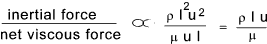This ratio is very important in fluid mechanics, mainly for problems involving flowing fluids, and it is called Reynolds number.  The ratio for dynamic similarity between two flows past geometrically similar boundaries and affected by only viscous and inertia forces is the same if the fluids have the same reynolds number.  In the UK for pipe flow studies the characteristic length( l ) is the diameter ( D ) and the characteristic velocity u is chosen as the mean velocity.

Dynamic similarity involving flow with gravity forces...
When considering forces with free surfaces e.g. flows over weirs, channel flows, or surface motion around ships, the most significant relationships is the ratio between the gravity forces and the inertia forces.   These are summerised below..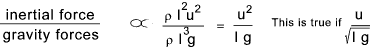This ratio u /( lg ) 1/2 is called the Froude number .   Dynamic similarity exists between two flows which involve fluids subject to only gravity and inertial forces if the Froude number , based on corresponding velocities and lengths, is the same for both fluids...

The primary dimensionless groups in Fluid mechanics are listed below.
 Group Name Represents Ratio of Forces Symbol ρ l u / μ Reynolds Number Inertia / Viscous Re u / ( lg ) 1/2 Froude Number Inertia / Gravity Fr u / ( lρ / γ )1/2 Weber Number Inertia / Surface Tension We u / a Mach Number Inertia / Elastic M

Note: a is identified as the local sonic velocity, with dimensions L .T -1

Simple Example

Consider a body moving with constant acceleration.  The relationship is expressed as ..... s = ut + at 2 /2
Expressing this in terms of dimensions..

Note....[ z ] is used to say the dimensions of z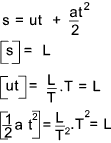The above examples simply illustrates that the equation is dimensionally correct.   This exercise can be continued to produce a non-dimensional equation.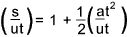The terms within the brackets are non dimensional groups which can be considered a single variables or groups.  These are generally called denoted using the symbol   Π the above equation can be expressed as

Π 1 = 1 + Π 2..... or.....Π 1 = F [ Π 2]

There is no real advantage in using the principle for this simple example but for more complex relationships the benefits can be significant.

Buckinghams Π theorem

Consider a physical phenomenon with an unknown defining equation.

First define what relationship is require. e.g The wind force experienced by a sphere

List the number of dependent variables and all relevant variables. eg F = f (d,u,ρ,μ )

Using base dimension (say L,M,T,η..F), set down the dimensions of all the variables.
e.g. F->[MLT-2] , d->[L] , u-> [LT-1]  , ρ ->; [ML-3] , μ ->[ML-1 T-1]

Count the number of variables (n = 5) count the number of base dimensions used to dimension the variables ( j = M,L,T).
note: For fluids j will generally = 3

Select j variables which include in their dimension which collectively include all the base dimensions (in this case M,L & T].
e.g. Choose d->[L], u-> [LT-1], and ρ ->; [ML-3]

Form k dimensionless groups [k = n - j = 2]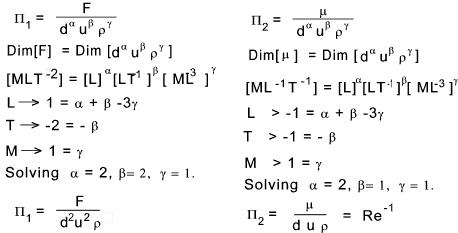Use the resulting dimensionless groups to establish a relationship in which one group which includes the dependent variable (F) as a function of the other groups..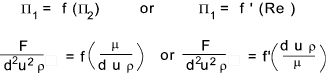Buckinghams's theorem simply states that if there is a relationship involving n variables and j base dimensions then k = n- j dimensionless groups ( Π groups ) can be created allowing physical relationships to be developed using experimental methods

 Useful Links Wikipedia Dimensional analysis.. Set of very useful and informative notes Dimensional Analysis -Tutorial Notes.. pdf Download very clear notes with exercises 1- Dimensional Analysis.. One of a number of lecture notes from Leed University An exploration of Physics by Dimensional Analysis.. A very eductional and informative set of notes

Fluids Index